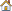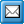﻿ SetFormula
QPR Knowledge Base 2017.1

SetFormulaDeveloper's Guide > QPR API > The Object Model > QPR Metrics > SCModel Commands:

SetFormulaSetFormulaRevision history:

Introduced in QPR 7.2.1

Last changed in QPR 2012

Sets formula for the specified element and series. Changes input type of this series to "Formula". All periods of the element are calculated.

Synopsis:

SCModel.SetFormula(ElementId, SeriesIdentifier, Formula)

Parameters:

ElementId: Integer

SeriesIdentifier: String. Must be identifier of some series that belongs to ElementId

Formula: String. Formula string like "AVERAGE(?.ACT())"

Required Rights:

Return Values:

Below are listed the return values that this function can return:

RV_SUCCESS

RV_MODEL_NOT_OPEN

RV_NO_RIGHTS

RV_ELEMENT_NOT_FOUND

RV_CANNOT_SET_INPUT_TYPE

RV_LOOP_IN_FORMULA

RV_OBJECT_IS_LOCKED

RV_UNKNOWN_ERROR

Example Procedure:

iRet = oModel.SetFormula(ElementId, "ACT", "1") 'set value 1 to all periods